# 6.1环境边界

## 6.1.1设置边界

stage.align = StageAlign.TOP_LEFT;
stage.scaleMode = StageScaleMode.NO_SCALE; 

private var left:Number = 0;
private var top:Number = 0;
private var right:Number = stage.stageWidth;
private var bottom:Number = stage.stageHeight;

OK，边界已经有了，用它们能做什么呢？判断所有移动的对象，看它们是否仍在这个空间内，这里可以使用 if 语句，简化的样式如下：

if(ball.x > stage.stageWidth) {
// do something
}
else if(ball.x < 0) {
// do something
}
if(ball.y > stage.stageHeight)
{
// do something
}
else if(ball.y < 0)
{
// do something
}

• 将对象移除；
• 重置到舞台上，像生成一个新对象一样(重置对象)；
• 重置到舞台上，将同一个对象放置在不同位置；
• 将其反弹回去。

## 6.1.2移除物体

if(ball.x > stage.stageWidth ||ball.x < 0 || ball.y > stage.stageHeight || ball.y < 0) {
removeChild(ball);
}

|| 符号意思是“或者”。这句话是说“如果物体超出了右边界，或左边界，或上边界，或下边界，就将它删除。”。 这里有一个小问题，也许大家现在还没有意识到，见图 6-1。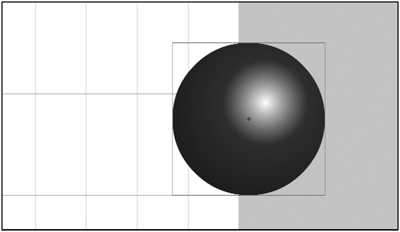if(ball.x - ball.radius > stage.stageWidth || ball.x + ball.radius < 0 || ball.y - ball.radius > stage.stageHeight || ball.y + ball.radius < 0) {
removeChild(ball);
}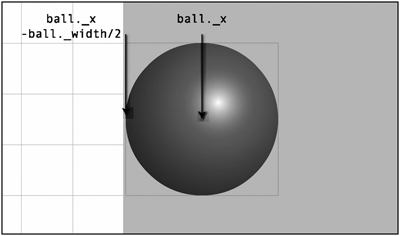package {
import flash.display.Sprite;

public class Ball extends Sprite {
private var color:uint;
public var vx:Number = 0;
public var vy:Number = 0;

this.color = color;
init();
}
public function init():void {
graphics.beginFill(color);
graphics.endFill();
}
}
}

package
{
import flash.display.Sprite;
import flash.display.StageAlign;
import flash.display.StageScaleMode;
import flash.events.Event;

public class Removal extends Sprite
{
private var count:int = 20;
private var balls:Array;

public function Removal()
{
init();
}

private function init():void
{
stage.scaleMode = StageScaleMode.NO_SCALE;
stage.align=StageAlign.TOP_LEFT;
balls = new Array();
for(var i:int = 0; i < count; i++)
{
var ball:Ball = new Ball(10);
ball.x = Math.random() * stage.stageWidth;
ball.y = Math.random() * stage.stageHeight;
ball.vx = Math.random() * 2 - 1;
ball.vy = Math.random() * 2 - 1;
balls.push(ball);
}
}

private function onEnterFrame(event:Event):void
{
for(var i:Number = balls.length - 1; i > 0; i--)
{
var ball:Ball = Ball(balls[i]);
ball.x += ball.vx;
ball.y += ball.vy;
if(ball.x - ball.radius > stage.stageWidth ||
ball.x + ball.radius < 0 ||
ball.y - ball.radius > stage.stageHeight ||
{
removeChild(ball);
balls.splice(i, 1);
if(balls.length <= 0)
{
removeEventListener(Event.ENTER_FRAME, onEnterFrame);
}
}

}
}
}
}

onEnterFrame 方法通过速度向量使小球移动，判断边界，并将出界的小球删除。请注意，不但要将小球从显示列表中删除，同时还要使用 Array.splice 函数将数组中的引用也删除。Array.splice 有两个参数：开始删除元素的索引，删除元素的个数。在这个例子中，只删除一个元素即当前索引处的元素。

for(var i:Number = balls.length - 1; i > 0; i--)

## 6.1.3重新生成物体

package
{
import flash.display.Sprite;
import flash.display.StageAlign;
import flash.display.StageScaleMode;
import flash.events.Event;

public class Fountain extends Sprite
{
private var count:int = 100;
private var balls:Array;
private var gravity:Number = 0.5;

public function Fountain()
{
init();
}

private function init():void
{
stage.scaleMode = StageScaleMode.NO_SCALE;
stage.align=StageAlign.TOP_LEFT;
balls = new Array();
for(var i:int = 0; i < count; i++)
{
var ball:Ball = new Ball(2, Math.random() * 0xffffff);
ball.x = stage.stageWidth / 2;
ball.y = stage.stageHeight;
ball.vx = Math.random() * 2 - 1;
ball.vy = Math.random() * -10 - 10;
balls.push(ball);
}
}

private function onEnterFrame(event:Event):void
{
for(var i:Number = 0; i < balls.length; i++)
{
var ball:Ball = Ball(balls[i]);
ball.vy += gravity;
ball.x += ball.vx;
ball.y += ball.vy;
if(ball.x - ball.radius > stage.stageWidth ||
ball.x + ball.radius < 0 ||
ball.y - ball.radius > stage.stageHeight ||
{
ball.x = stage.stageWidth / 2;
ball.y = stage.stageHeight;
ball.vx = Math.random() * 2 - 1;
ball.vy = Math.random() * -10 - 10;
}

}
}
}
}

## 6.1.4屏幕折回

package
{
import flash.display.Sprite;
import flash.events.Event;
import flash.events.KeyboardEvent;
import flash.ui.Keyboard;
import flash.display.StageAlign;
import flash.display.StageScaleMode;

public class ShipSim2 extends Sprite
{
private var ship:Ship;
private var vr:Number = 0;
private var thrust:Number = 0;
private var vx:Number = 0;
private var vy:Number = 0;

public function ShipSim2()
{
init();
}

private function init():void
{
stage.scaleMode = StageScaleMode.NO_SCALE;
stage.align=StageAlign.TOP_LEFT;
ship = new Ship();
ship.x = stage.stageWidth / 2;
ship.y = stage.stageHeight / 2;
}

private function onKeyDownHandler(event:KeyboardEvent):void
{
switch(event.keyCode)
{
case Keyboard.LEFT :
vr = -5;
break;

case Keyboard.RIGHT :
vr = 5;
break;

case Keyboard.UP :
thrust = 0.2;
ship.draw(true);
break;

default :
break;
}
}

private function onKeyUpHandler(event:KeyboardEvent):void
{
vr = 0;
thrust = 0;
ship.draw(false);
}

private function onEnterFrame(event:Event):void
{
ship.rotation += vr;
var angle:Number = ship.rotation * Math.PI / 180;
var ax:Number = Math.cos(angle) * thrust;
var ay:Number = Math.sin(angle) * thrust;
vx += ax;
vy += ay;
ship.x += vx;
ship.y += vy;
var left:Number = 0;
var right:Number = stage.stageWidth;
var top:Number = 0;
var bottom:Number = stage.stageHeight;
if (ship.x - ship.width / 2 > right)
{
ship.x = left - ship.width / 2;
}
else if (ship.x + ship.width / 2 < left)
{
ship.x = right + ship.width / 2;
}
if (ship.y - ship.height / 2 > bottom)
{
ship.y = top - ship.height / 2;
}
else if (ship.y < top - ship.height / 2)
{
ship.y = bottom + ship.height / 2;
}
}
}
}

## 6.1.5反弹

if(ball.x – ball.radius > right) . . .

if(ball.x + ball.radius > right) . . .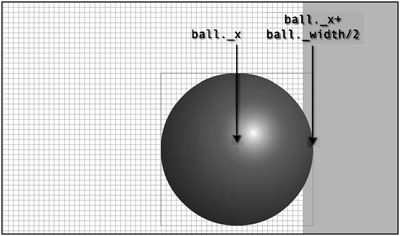if(ball.x + ball.radius > right) {
vx *= -1;
}
else if(ball.x – ball.radius < left) {
vx *= -1;
}

• 判断物体是否超出了边界；
• 如果是，将其置到边界处；
• 然后将它的速度向量取反。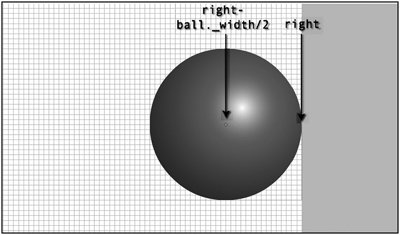package
{
import flash.display.Sprite;
import flash.display.StageAlign;
import flash.display.StageScaleMode;
import flash.events.Event;

public class Bouncing extends Sprite
{
private var ball:Ball;
private var vx:Number;
private var vy:Number;

public function Bouncing()
{
init();
}

private function init():void
{
stage.scaleMode = StageScaleMode.NO_SCALE;
stage.align=StageAlign.TOP_LEFT;

ball = new Ball();
ball.x = stage.stageWidth / 2;
ball.y = stage.stageHeight / 2;
vx = Math.random() * 10 - 5;
vy = Math.random() * 10 - 5;
}

private function onEnterFrame(event:Event):void
{
ball.x += vx;
ball.y += vy;
var left:Number = 0;
var right:Number = stage.stageWidth;
var top:Number = 0;
var bottom:Number = stage.stageHeight;

{
vx *= -1;
}
else if(ball.x - ball.radius < left)
{
vx *= -1;
}
{
vy *= -1;
}
else if(ball.y - ball.radius < top)
{
vy *= -1;
}
}
}
}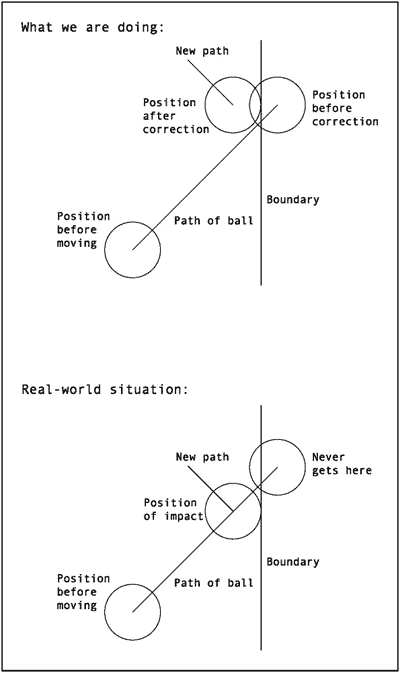private var bounce:Number = -0.7;

2006 - 2023，推荐分辨率1024*768以上，推荐浏览器Chrome、Edge等现代浏览器，截止2021年12月5日的访问次数：1872万9823 站长邮箱